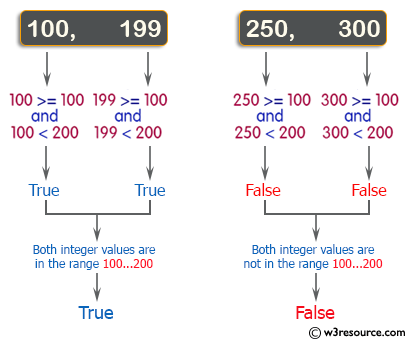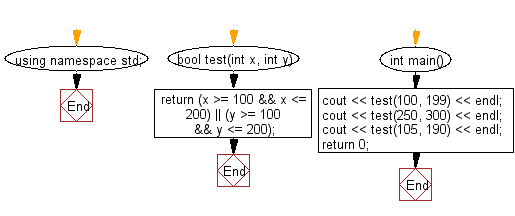﻿ C++ : Check two integers, if either of them is in a range# C++ Exercises: Check two given integers whether either of them is in the range 100..200 inclusive

## C++ Basic Algorithm: Exercise-14 with Solution

Write a C++ program to check two given integers whether either of them is in the range 100..200 inclusive.

Sample Solution:

C++ Code :

``````#include <iostream>
using namespace std;

bool test(int x, int y)
{
return (x >= 100 && x <= 200) || (y >= 100 && y <= 200);
}

int main()
{
cout << test(100, 199) << endl;
cout << test(250, 300) << endl;
cout << test(105, 190) << endl;
return 0;
}
``````

Sample Output:

```1
0
1
```

Pictorial Presentation:Flowchart:C++ Code Editor:

Contribute your code and comments through Disqus.

What is the difficulty level of this exercise?

﻿